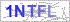## Praat脚本编程中文教程：认识数值变量与字符串变量

标签：Praat脚本,Praat中文教程,Praat编程
修改 | 阅读（1843）| 评论（0）

```n=1234
n1=5678
n_a=9
n_b=0```

```n=1234
writeInfoLine:n```

`1234`

```n=1234
writeInfoLine:n
n=5678
appendInfoLine:n```

```1234
5678```

```n=1234
writeInfoLine:"n的值为:",n
n=5678
appendInfoLine:"n的值变为:",n```

```n的值为:1234
n的值变为:5678```

```n1=2
n2=n1*3
n3=4+5
n1=n3
writeInfoLine:"n1的值为:",n1
appendInfoLine:"n2的值为:",n2
appendInfoLine:"n3的值为:",n3```

```n1的值为:9
n2的值为:6
n3的值为:9```

```text1\$="Hello"
text2\$="World"
writeInfoLine:text1\$+" "+text2\$```

`Hello World`

```a=1
b=2
c\$="1"
d\$="2"
writeInfoLine:a+b
appendInfoLine:c\$+d\$```

```3
12```

`writeInfoLine:a+c\$`

`writeInfoLine:a,c\$`

` 11`

QQ登录(无需注册直接登录可进行回复)